# Cohn-Vossen transformation

(diff) ← Older revision | Latest revision (diff) | Newer revision → (diff)
A correspondence between a pair of isometric surfacesandand an infinitesimal deformation of the so-called mean surface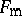: If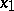and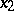are the radius (position) vectors of the surfacesand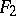, then the radius vector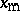ofis given by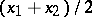, and the field of velocitiesof the infinitesimal deformation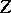is. It was introduced by S.E. Cohn-Vossen . Ifandare smooth surfaces and if the angle between the semi-tangents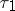andto the curves on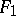andcorresponding under the isometry is less than, thenturns out to be smooth. This fact has enabled one to reduce in a number of cases the study of the isometry ofandto the study of infinitesimal deformations (cf. Infinitesimal deformation) of. For fixed pointsonandonthe Cohn-Vossen transformation defines a Cayley transformation of the orthogonal matrix, representing the isometry of the tangent space toto that of, into a skew-symmetric matrix describing the infinitesimal deformation of.